﻿ 基于势流理论的大型船舶最小装机功率波浪增阻预报
 舰船科学技术2020, Vol. 42Issue (8): 88-92    DOI: 10.3404/j.issn.1672-7649.2020.08.016PDF

Calculation of wave added thrust of large ships concerning minimum propulsion requirement based on potential flow theory
WU Si-ying, WU Dan, DING Jing-lei
Shanghai Waigaoqiao Shipbuilding Co., Ltd., Shanghai 200137, China
Abstract: IMO puts forward strict requirements for minimum propulsion of ships. In order to obtain minimum power required by standard, it is necessary to calculate the total resistance of ships under extreme sea conditions, among which the calculation of wave added thrust is the most difficult. At present, the CFD method is widely used in calculating wave added thrust of ships, however when it comes to large ships like VLCC the method is not applicable. In order to calculate wave added thrust of VLCC quickly and accurately, this paper takes a 320 000 ton VLCC as an example, calculates the resistance of VLCC under static water and wave environment by software Aqwa, subtracting the former by the latter. Compared with the model test results, it is found that the three-dimensional potential flow theory is efficient and accurate in predicting wave added thrust, which can be used as a reference for the prediction of wave added thrust in the early stage of development of such ships. However, it is not recommended to adopt this method because of the large error in the calculation of resistance in still water and waves. This paper only verifies the accuracy of this method at low Frude number, and whether this method is effective at high Frude number needs further verification.
Key words: minimum propulsion power     wave added thrust     three-dimensional potential flow theory     VLCC
0 引　言

1 计算方法

3种坐标系相互关系如图1所示。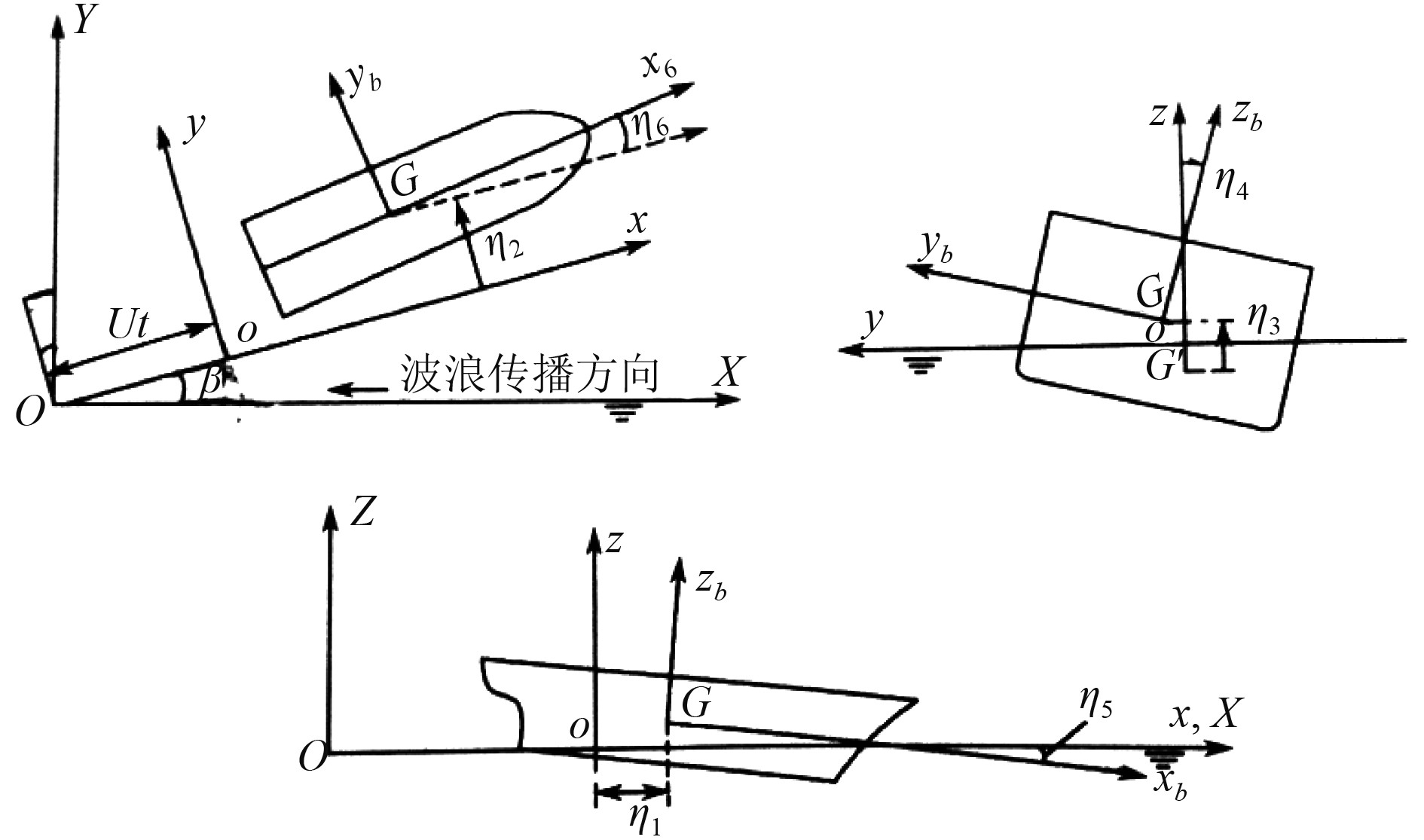图 1 描述船体运动的3个坐标系 Fig. 1 Three coordinate system for describing the movement of the ship

 ${\nabla ^2}\phi = \frac{{{\partial ^2}\phi }}{{\partial {x^2}}} + \frac{{{\partial ^2}\phi }}{{\partial {y^2}}} + \frac{{{\partial ^2}\phi }}{{\partial {z^2}}} = 0 \;{\text{，}}$ (1)

 $\frac{{\partial \phi }}{{\partial z}} = 0,z = - d\;{\text{，}}$ (2)

 $\frac{{\partial \phi }}{{\partial n}} = {V_n}\;{\text{，}}$ (3)

 $\frac{{\partial \eta }}{{\partial t}} + u\frac{{\partial \eta }}{{\partial x}} + v\frac{{\partial \eta }}{{\partial y}} - \frac{{\partial \phi }}{{\partial z}} = 0,z = \eta (x,y,t)\;{\text{。}}$ (4)

 $p = - \rho \frac{{\partial \phi }}{{\partial t}}\;{\text{，}}$ (5)

 ${F_i}\left( t \right) = - \iint\limits_{{S_0}} {pn{\rm{d}}S} = - \iint\limits_{{S_0}} {\rho \frac{{\partial \phi }}{{\partial t}}{n_i}}{\rm{d}}s\;{\text{，}}$ (6)

 ${{F}_{i}}\left( t \right)=-\iint\limits_{{{S}_{0}}}{p\left( r\cdot n \right){\rm{d}}S}=-\iint\limits_{{{S}_{0}}}{\rho \frac{\partial \phi }{\partial t}(r\cdot {{n}_{i}})}{\rm{d}}s\;{\text{。}}$ (7)

2 模型试验 2.1 船舶主尺度表 1 主尺度参数 Tab.1 Main particulars
2.2 船体模型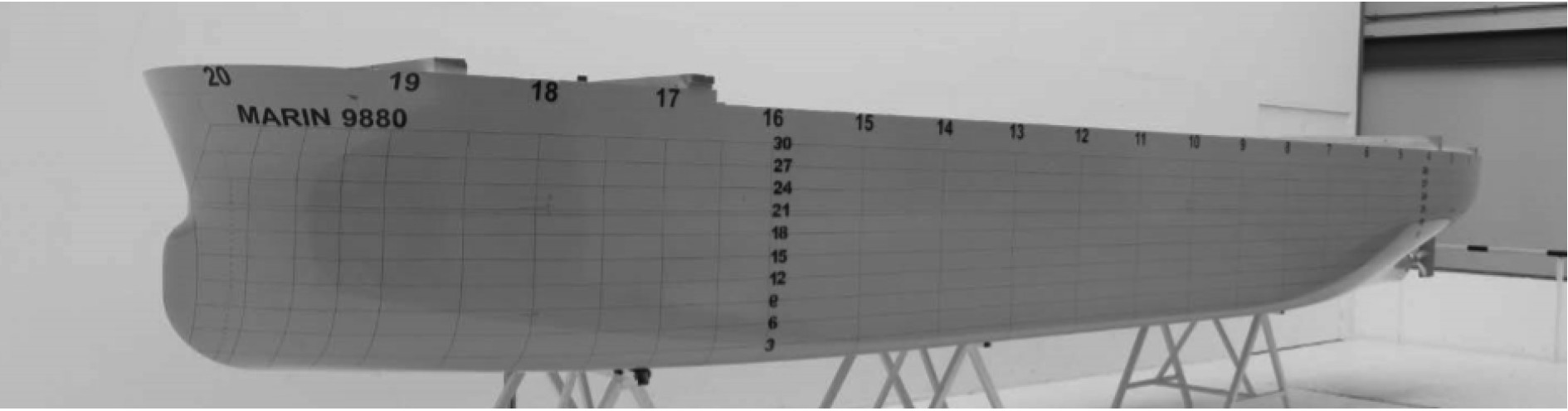图 2 船体模型 Fig. 2 Ship modle表 2 波浪增阻试验结果 Tab.2 Testing Results of the Added Resistance

2.3 试验工况及结果

 ${V_{{S}}} = \max \left( {4{\rm{kn}},{V_{{\rm{kn}}}} = {V_{{\rm{ref}}}} - 10.0 \times \left( {{A_{\rm{R}}}\% - 0.9\% } \right)} \right)\;{\text{，}}$ (8)
 ${A_{\rm{R}}}\% = {A_{\rm{R}}}/{A_{{\rm{LS,COR}}}} \cdot 100\% \;{\text{，}}$ (9)
 ${A_{{\rm{LS,COR}}}} = {L_{{\rm{PP}}}}{T_{\rm{m}}}(1.0 + 25.0 \times {({B_{{\rm{WL}}}}/{L_{{\rm{PP}}}})^2})\;{\text{。}}$ (10)

3 数值计算 3.1 模型建立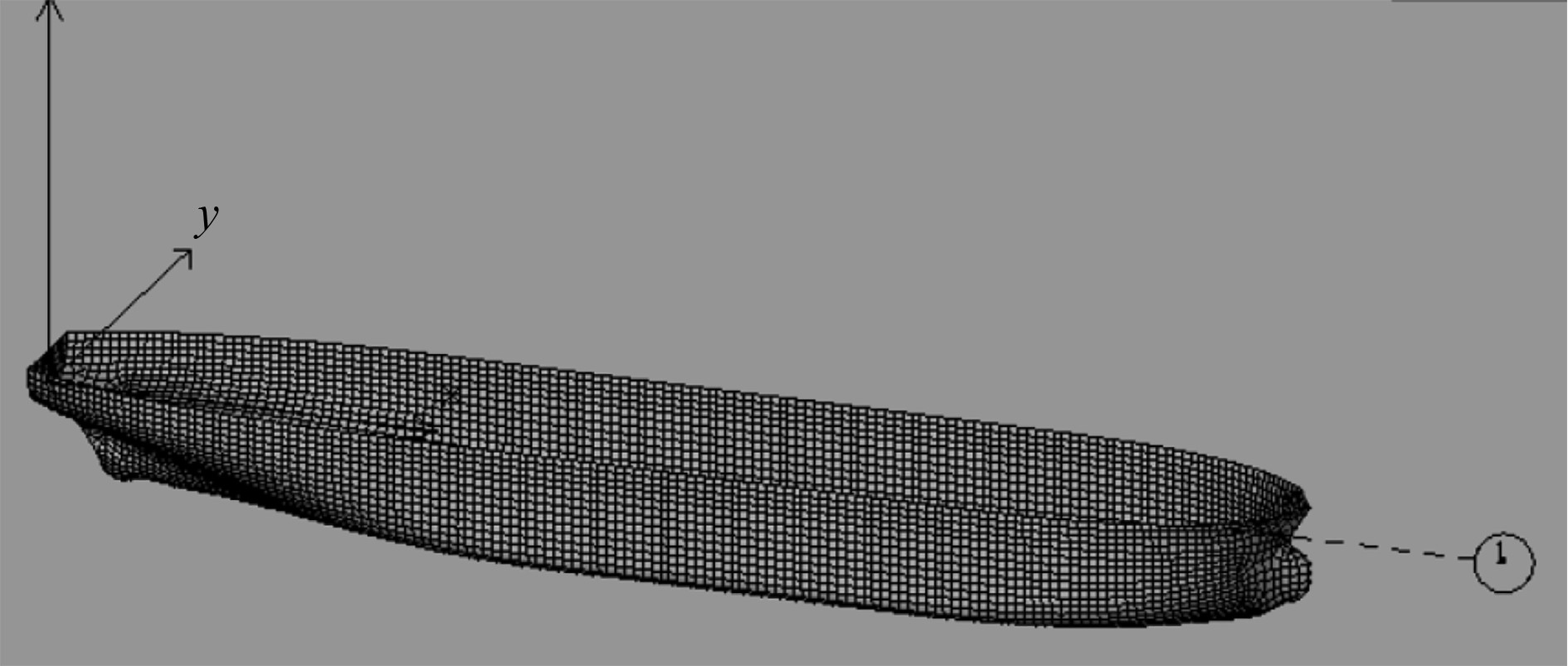图 3 船体网格模型 Fig. 3 Ship mesh model表 3 波浪增阻数值模拟结果 Tab.3 Numerical Simulation Results of the Added Resistance
3.2 数值计算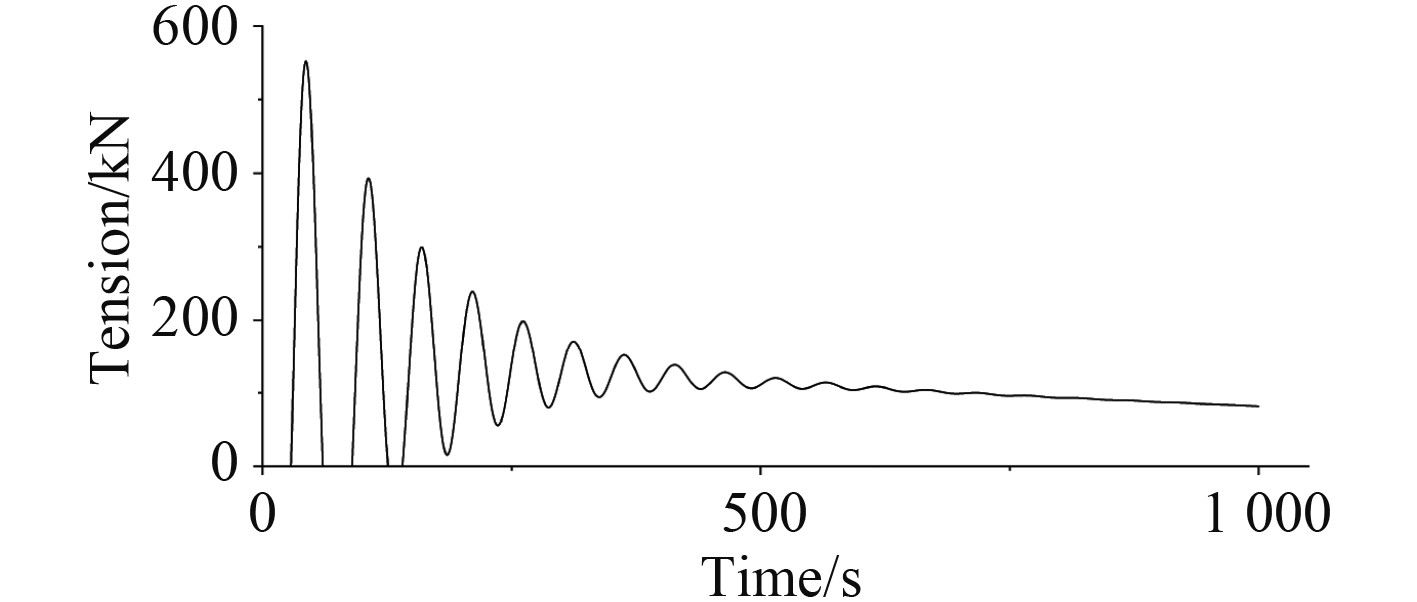图 4 静水条件下拖缆张力时程曲线 Fig. 4 Time history of towline tension in calm water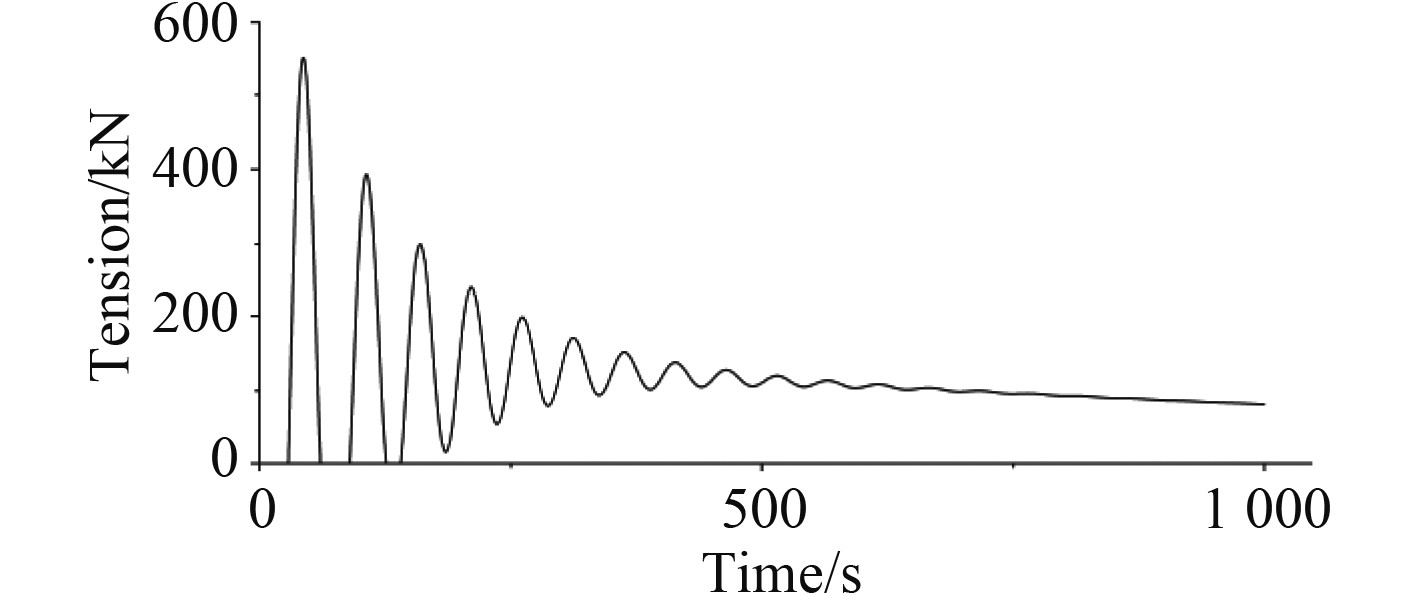图 5 波浪条件下拖缆张力时程曲线（ω=0.9） Fig. 5 Time history of towline tension in wave

3.3 计算结果

4 数值计算与试验结果对比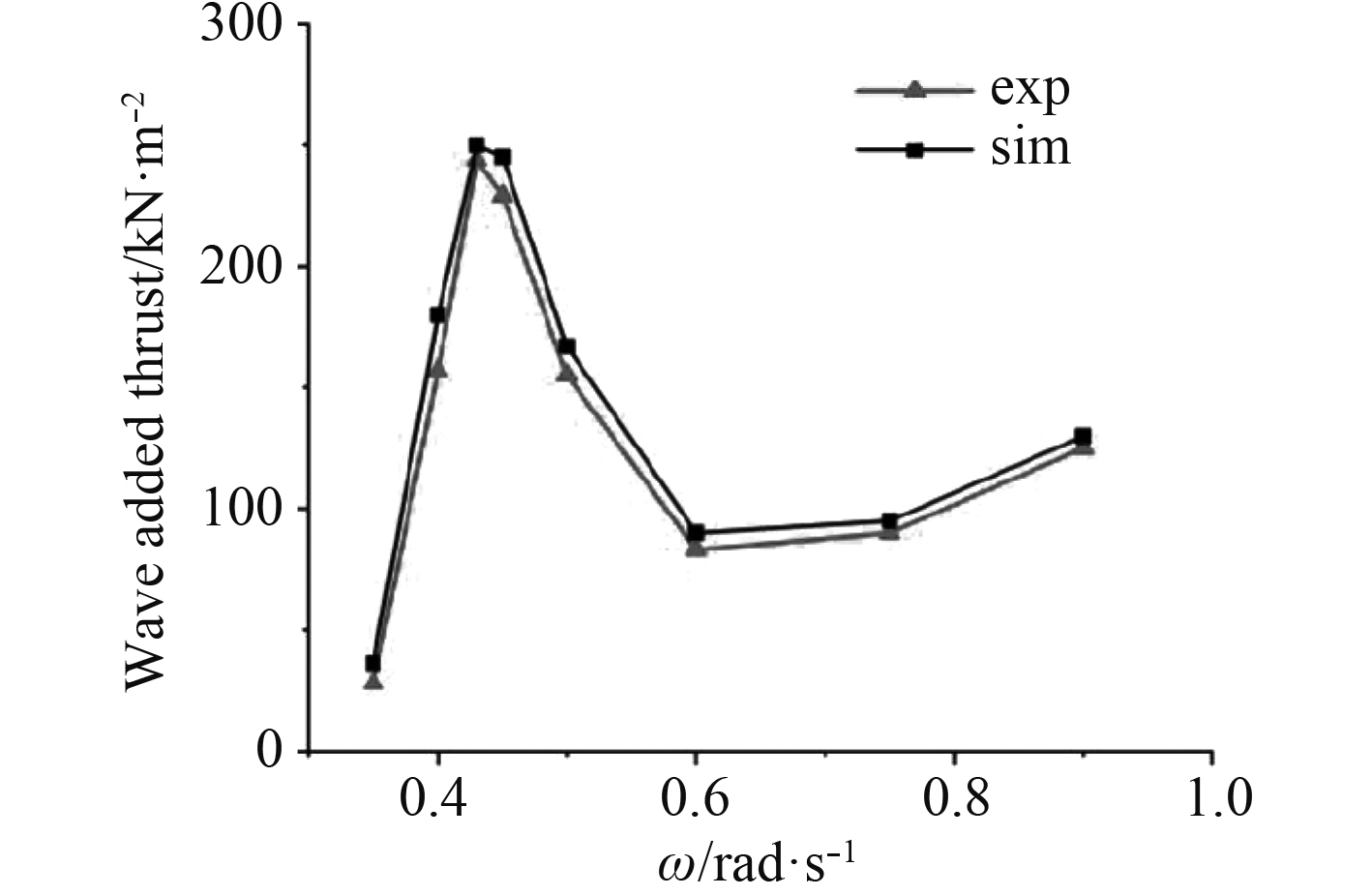图 6 数值模拟与模型试验结果对比 Fig. 6 Results Comparision between Numerical Simulation and Model Test

5 结　语

1）采用三维势流理论预报波浪增阻计算效率高且与模型试验得到的结果规律性一致，整体误差较小。因此，这一计算方法可作为此类大型船舶波浪增阻预报的有效手段。

2）虽然波浪增阻数值误差较小，但这一理论计算得出的静水或波浪中航行阻力与试验值相比误差较大，主要原因是未能考虑到摩擦阻力及粘压阻力，因此单独计算静水阻力或波浪总阻力时不建议采用这一方法。

3）试验仅考虑了航速5.27 kn的波浪增阻，能够满足船厂开发初期用于预估航速及验证满足最小功率要求。但当航速增加时非线性效应必然越发明显，占阻力比重也会增加。因此，这一方法是否适用于计算高Frude数时的波浪增阻计算还需进一步验证。

  International organization for standardization. Ship and marine technology — guidelines for the assessment of speed and power performance by analysis of speed trial data[S]. 2015.  MEPC. 232 (65), 2013. Interim guidelines for determining minimum propulsion power to maintain the manoeuvrability of ship in adverse conditions[S].  魏锦芳, 陈京普, 王杉, 等. 船舶全浪向波浪增阻数值计算与模型试验验证[J]. 中国造船, 2017, 58(4): 24-30. DOI:10.3969/j.issn.1000-4882.2017.04.003  于海, 封培元, 熊小青, 等. 中型豪华游船波浪增阻数值模拟分析[J]. 船舶, 2019, 30(1): 99-104. DOI:10.3969/j.issn.1001-9855.2019.01.018  李纳, 谌志新. 50 m围网渔船波浪增阻数值模拟[J]. 船舶工程, 2019, 41(4): 7-12.  尹崇宏, 吴建威, 万德成. 基于IDDES方法的模型尺度和实尺度VLCC阻力预报与流场分析[J]. A辑, 2016, 31(3): 259-268.  HU Jinpeng, ZHU Ling, LIU Shihui. Analysis and optimization on the flow ability of wave buoy based on AQWA[J]. IOP Conference Series: Earth and Environmental Science, 2018, 171(1).  FALTINSEN. O. M. 船舶与海洋工程环境荷载[M]. 上海: 上海交通大学出版社, 2008: 8-9.  吴思莹. LNG-FSRU船舶在波浪中的运动与液舱内流体晃荡的耦合数值分析[D]. 镇江: 江苏科技大学, 2016.